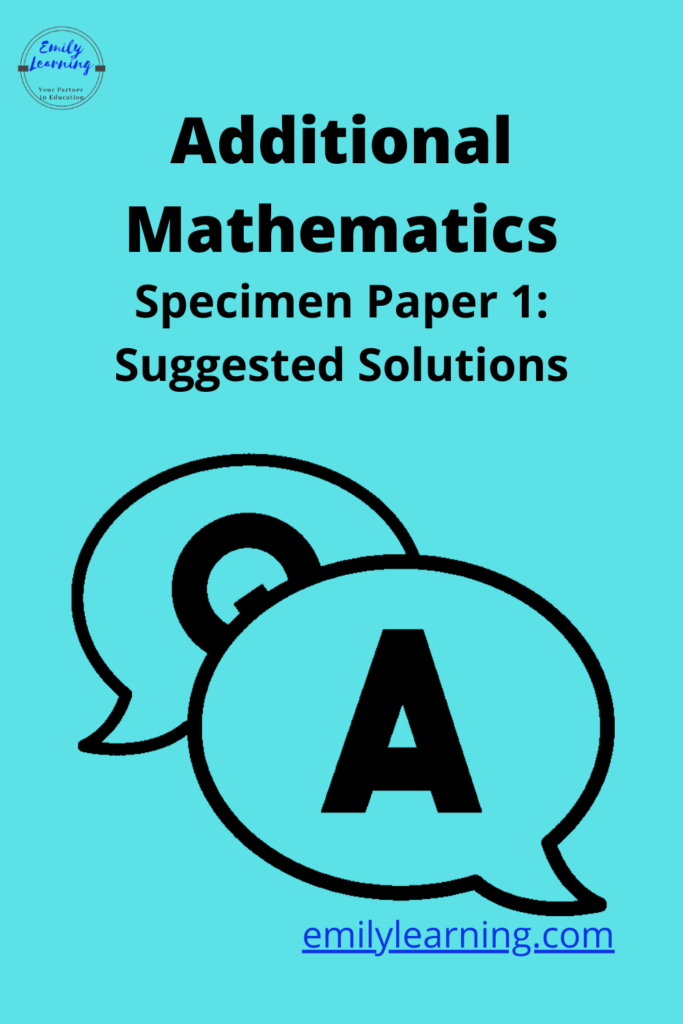Here, you will find the suggested solutions to Add Math Specimen Paper 1. The specimen paper can be downloaded from the Singapore Examinations and Assessment Board (SEAB) website. The link to the 2020 specimen paper 1 can be found here.

Question 1: Coordinate Geometry and solving simultaneous equations

In this question, students are tested on the equations of a straight line from the coordinate geometry chapter. As there are 2 unknowns, these could be found by solving simultaneous equations.

For worked solutions for question 1, watch the video here:

Question 2: Sketching of Trigonometric Curves

Trigonometry is a big topic for O Level Additional Mathematics. You are tested on solving equations, sketching, R- formula, proving identities. In this question, you are tested on sketching trigonometric curves.

### Question 3: Binomial Expansion

This question is on binomial expansion. There are two types of questions on binomial expansion, one that requires you to expand (up to a certain term) and another type requires you to use the term formula to find a particular term. I would say that this question is mainly on expansion. Even part (b) which asks you to find the term independent of involves students doing algebraic expansion instead of using the binomial’s term formula.

### Question 4: Differentiation (increasing and decreasing function and rate of change)

Differentiation is a big topic in O Level Additional Mathematics. In fact, differentiation is the largest topic in O Level Add Maths. This question tests increasing and decreasing function in part (a) and rate of change (using chain rule) in part (b).

### Question 5: Exponential and Natural Logarithm

This question on exponential and natural logarithm is relatively simple. You just need to substitute the values given and find the unknown. While the question is an exponential question, you will need to convert the exponent to logarithm to solve the unknowns.

### Question 6: Integration

This question is on integration. You are given an expression of the second derivative, and you are asked to find y. To do so, you need to integrate the expression twice.

This question is an interesting one. You are asked to complete the square of the quadratic expression in part (a). For part (b), you are to show that the two curves do not intersect, not by using discriminant, but rather by finding the maximum and minimum point from the complete the square form in part (a). Well, you could prove that the curves do not intersect using the discriminant method, but because the question asks you to make use of your answer from (a), you need to use the maximum and minimum point to prove that they do not intersect.

### Question 8: Trigonometric (special angles) and Surds

At first glance, this question seems like a trigonometric question, testing students on the special angles and trigonometric identity. But actually, it is a combination of trigonometry and surds (simplying surds and rationalising the denominator containing surds).

### Question 9: Differentiation (Maximum and Minimum)

This is not the first time we see the differentiation topic in the same paper. Question 4 is also on differentiation. But unlike question 4 which tests students on increasing functions and rate of change (using chain rule), question 9 is on the application of differentiation to problems involving maximum and minimum.

### Question 10: Factor and Remainder Theorem and Solving of Cubic Equation

This question is from the chapter on polynomials. In part (a) students are tested on the factor and remainder theorem concept. In part (b), students are tested on solving the cubic equation, given a linear factor.

### Question 11: Proofs in Plane Geometry

This is a question involving proofs in plane geometry. In particular, we use concepts such as alternate angles (if you see parallel lines, alternate angles or corresponding angles should come to your mind!), alternate segment theorem (since you have a tangent to a circle), and cyclic quadrilateral.

### Question 12: Trigonometric identities and solving trigonometric equations

This question tests concepts from the Trigonometry topic. In part (a), students make use of trigonometry identities to prove the given identity. In part (b) is all about solving, while in part (c), students make use of double angle formula to solve the given equation.

### Question 13: Application of differentiation and integration to displacement, velocity, and acceleration questions

This question is on the application of differentiation and integration (calculus) to displacement, velocity and accleration. Basically, it is a kinematics question. You are given an equation involving velocity (v), and need to convert this to acceleration (a) in part (b) of the question. To do so, you’ll differentiate velocty. In part (c) of the question, you are asked about displacement, so you’ll need to integrate velocity to get displacement.

## Learn On-demand – A- Math Courses

If you want to learn a particular topic in detail, check out our on-demand O Level A Math courses here.

Suggested Answers for other A Math Papers:

1.Sz

Hi Emily,

I think you may want to re-look at your answers for Q7 & Q10 for Specimen Paper 1.

Also for Q13(c), the answer should be rounded to 3 sig figs, so it should be 108m.

Thanks,
Sz

•Emily

Hi SZ.

Thanks for the comment. Made the changes for 7 and 10. For question 13c, since 108 1/3 is the exact answer, we do not need to round off to 3 significant figures for the A Math exam.

2.AH

Hi ma’am,
I think that for qn 13(b) the answer should be 1.7m/s squared since (0.2)(6)+0.5= 1.7 and not 0.8

•Emily

Hi AH, thanks for pointing that out! Changes made.